# DFA Solved Examples | How to Construct DFA

## Construction Of DFA-

Before you go through this article, make sure that you have gone through the previous article on Type-01 Problems.

## Type-02 Problems-

In Type-02 problems, we will discuss the construction of DFA for languages consisting of strings starting with a particular substring.

## Steps To Construct DFA-

Following steps are followed to construct a DFA for Type-02 problems-

### Step-01:

• Determine the minimum number of states required in the DFA.
• Draw those states.

Use the following rule to determine the minimum number of states-

### RULE

Calculate the length of substring.

All strings starting with ‘n’ length substring will always require minimum (n+2) states in the DFA.

### Step-02:

• Decide the strings for which DFA will be constructed.
• The method for deciding the strings has been discussed in this Video.

### Step-03:

• Construct a DFA for the strings decided in Step-02.

Remember the following rule while constructing the DFA-

### RULE

While constructing a DFA,

• Always prefer to use the existing path.
• Create a new path only when there exists no path to go with.

### Step-04:

• Send all the left possible combinations to the dead state.
• Do not send the left possible combinations over the starting state.

## Problem-01:

Draw a DFA for the language accepting strings starting with ‘ab’ over input alphabets ∑ = {a, b}

## Solution-

Regular expression for the given language = ab(a + b)*

### Step-01:

• All strings of the language starts with substring “ab”.
• So, length of substring = 2.

Thus, Minimum number of states required in the DFA = 2 + 2 = 4.

It suggests that minimized DFA will have 4 states.

### Step-02:

We will construct DFA for the following strings-

• ab
• aba
• abab

### Step-03:

The required DFA is-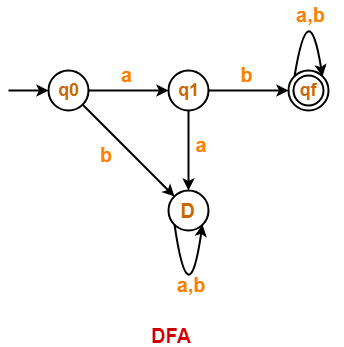## Problem-02:

Draw a DFA for the language accepting strings starting with ‘a’ over input alphabets ∑ = {a, b}

## Solution-

Regular expression for the given language = a(a + b)*

### Step-01:

• All strings of the language starts with substring “a”.
• So, length of substring = 1.

Thus, Minimum number of states required in the DFA = 1 + 2 = 3.

It suggests that minimized DFA will have 3 states.

### Step-02:

We will construct DFA for the following strings-

• a
• aa

### Step-03:

The required DFA is-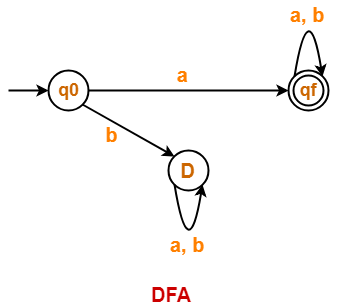## Problem-03:

Draw a DFA for the language accepting strings starting with ‘101’ over input alphabets ∑ = {0, 1}

## Solution-

Regular expression for the given language = 101(0 + 1)*

### Step-01:

• All strings of the language starts with substring “101”.
• So, length of substring = 3.

Thus, Minimum number of states required in the DFA = 3 + 2 = 5.

It suggests that minimized DFA will have 5 states.

### Step-02:

We will construct DFA for the following strings-

• 101
• 1011
• 10110
• 101101

### Step-03:

The required DFA is-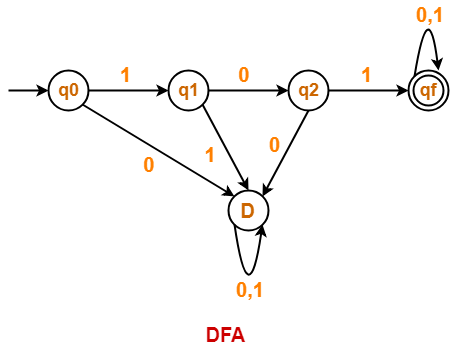## Problem-04:

Draw a DFA that accepts a language L over input alphabets ∑ = {0, 1} such that L is the set of all strings starting with ’00’.

## Solution-

Regular expression for the given language = 00(0 + 1)*

### Step-01:

• All strings of the language starts with substring “00”.
• So, length of substring = 2.

Thus, Minimum number of states required in the DFA = 2 + 2 = 4.

It suggests that minimized DFA will have 4 states.

### Step-02:

We will construct DFA for the following strings-

• 00
• 000
• 00000

### Step-03:

The required DFA is-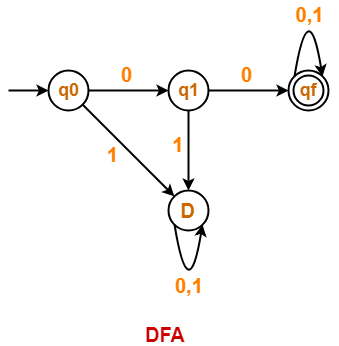## Problem-05:

Construct a DFA that accepts a language L over input alphabets ∑ = {a, b} such that L is the set of all strings starting with ‘aa’ or ‘bb’.

## Solution-

Regular expression for the given language = (aa + bb)(a + b)*

### Step-01:

Minimum number of states required in the DFA = 5.

It suggests that minimized DFA will have 5 states.

### Step-02:

We will construct DFA for the following strings-

• aa
• aaa
• aaaa
• bb
• bbb
• bbbb

### Step-03:

The required DFA is-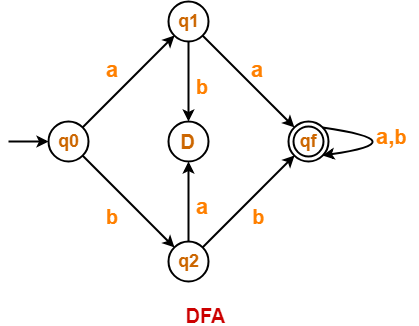## Problem-06:

Construct a DFA that accepts a language L over input alphabets ∑ = {a, b} such that L is the set of all strings starting with ‘aba’.

## Solution-

Regular expression for the given language = aba(a + b)*

### Step-01:

• All strings of the language starts with substring “aba”.
• So, length of substring = 3.

Thus, Minimum number of states required in the DFA = 3 + 2 = 5.

It suggests that minimized DFA will have 5 states.

### Step-02:

We will construct DFA for the following strings-

• aba
• abaa
• abaab
• abaaba

### Step-03:

The required DFA is-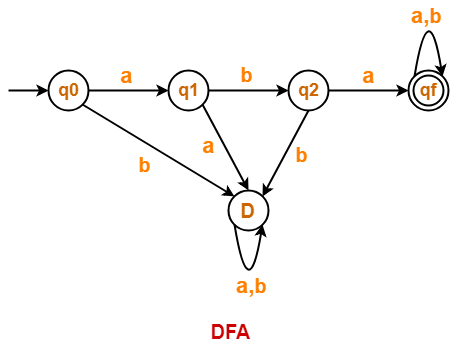Also Read- Converting DFA to Regular Expression

To gain better understanding about Construction of DFA,

Watch this Video Lecture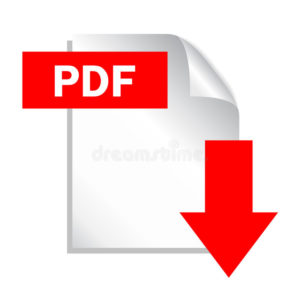Next Article- Minimization of DFA

Get more notes and other study material of Theory of Automata and Computation.

Watch video lectures by visiting our YouTube channel LearnVidFun.

SummaryArticle Name
DFA Solved Examples | How to Construct DFA
Description
How to construct DFA- This article discusses construction of DFA with examples. DFA Solved Examples. DFA Construction Problems. Practice Problems based on Construction of DFA.
Author
Publisher Name
Gate Vidyalay
Publisher Logo